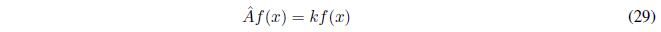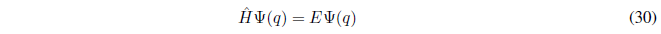Eigenvalue equations are those that have the following form:The operator $\hat{A}$ acts on the function f(x) and generates the function multiplied by a constant k. It is an eigenvalue equation. f(x) is the eigenfunction of the operator $\hat{A}$ and k is the eigenvalue. Eigenvalue equations are of great importance in Quantum Mechanics since the Schrödinger equation is such an equation.It is simple to show that if a function f(x) is proper to an operator $\hat{A}$ with eigenvalue k, then all functions of the form cf(x), where c is a constant, are proper to the operator $\hat{A}$ with eigenvalue k.